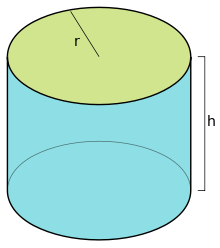# Cylinder 47923

The tank has the shape of a rotating cylinder with a base diameter d = 3.4 m and a height of 4.5 m.
How many liters of water are in the tank if the tank is filled to 2/3?

V =  27237.6083 l

### Step-by-step explanation:Did you find an error or inaccuracy? Feel free to write us. Thank you!

Tips for related online calculators
Need help calculating sum, simplifying, or multiplying fractions? Try our fraction calculator.
Do you know the volume and unit volume, and want to convert volume units?